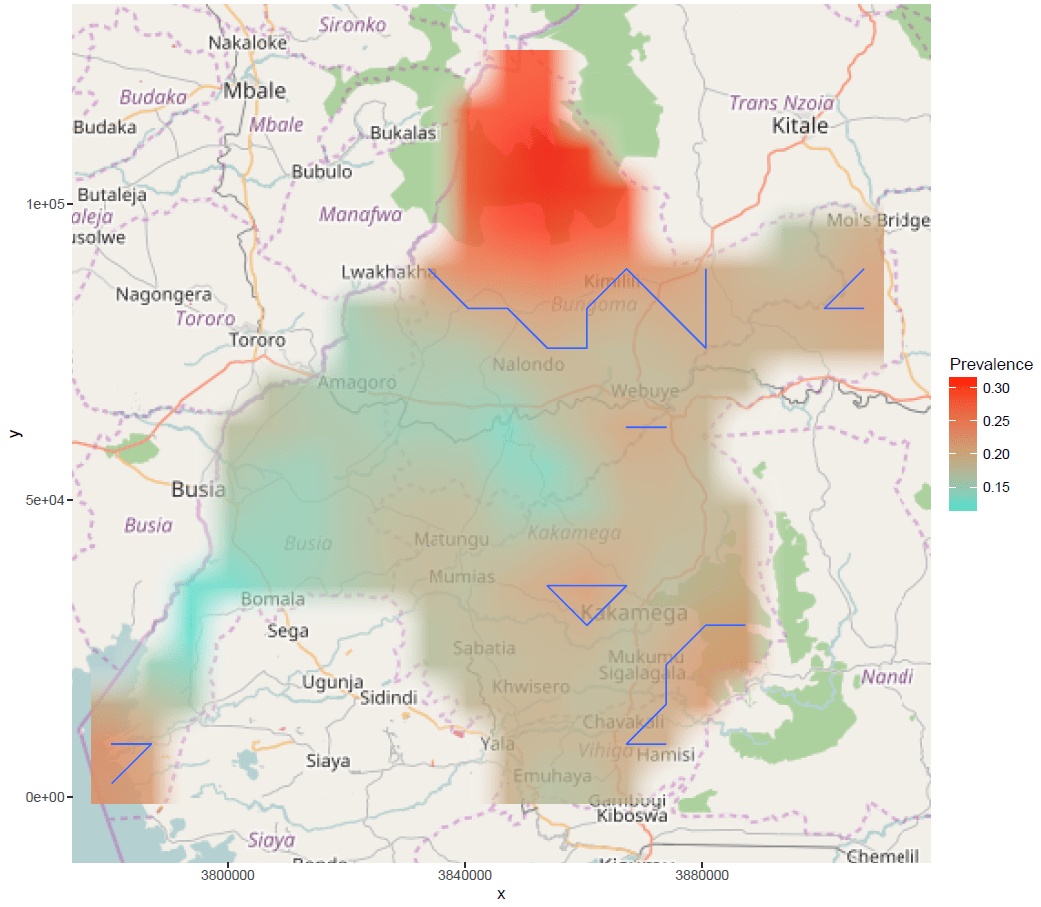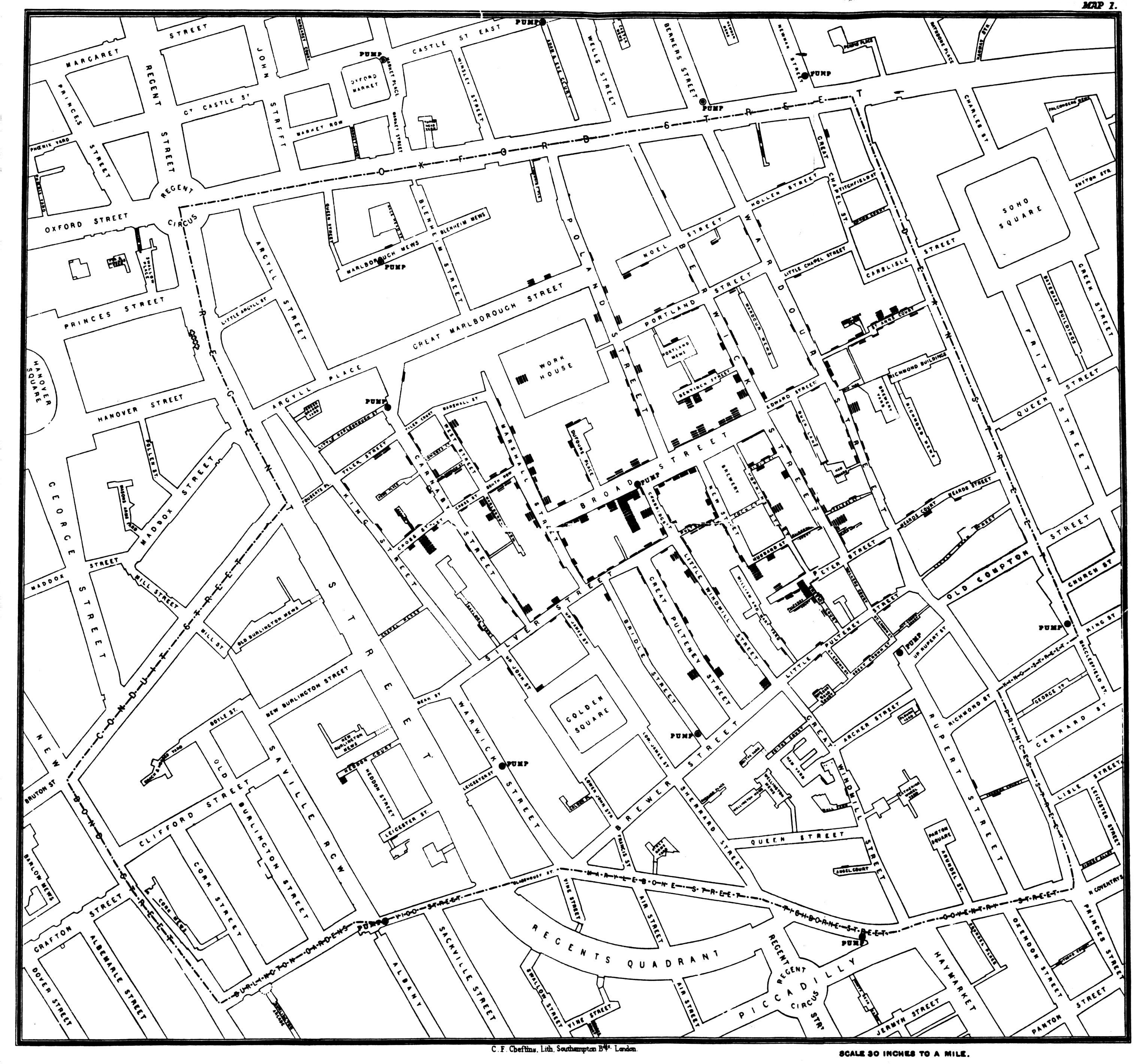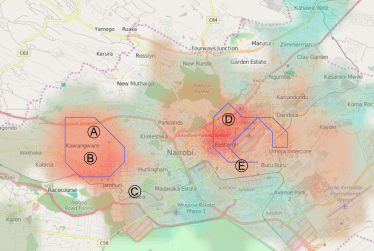# Geostatistical modelling with R and Stan

One of the advantages of writing blogs is that it can help to refresh and consolidate you thoughts on a topic. And when you spend a lot of time writing stats code, other people’s blogs that discuss how to code specific statistical models can be invaluable. I have recently found myself delving into spatial modelling and geostatistics with the aim of taking survey data from a number of discrete locations to model an underlying continuous distribution. This is typically for something like disease prevalence; the map featured in our post about slum health is an example. However, I have wanted to code my own Bayesian geostatistical model. There is a wealth of literature on these models: we recently featured one on a journal round up. But, moving from these models to usable code is not always obvious, so this post outlines an example using the example of mapping childhood stunting in Kenya with data from the Kenya Demographic and Health Survey.

## A geostatistical model

A geostatistical model, in its most basic form, analyses spatially discrete data sampled across an area that are assumed to be sampled from some underlying and unobserved continuous process. We often want to model this continuous process to be able to predict outcomes at a new location.

The survey locations across a given area,$A$, can be denoted as$x_i \in A \subset \mathbb{R}^2$ for locations$i=1,...,n$. At each location there is an underlying prevalence or probability,$p(x_i)$, the number of sampled individuals or households,$m_i$ and the observed outcomes$Y_i$. The standard sampling model is then binomial:$Y_i \sim Bin(m_i,p(x_i))$

To make inferences about the distribution of the prevalence of a disease or probability of some outcome across the area and to predict the prevalence at new locations, we need to model the link between the$p(x_i)$ at different locations. The following logistic regression model is often used:$log\frac{p(x_i)}{1-p(x_i)} = z(x_i)'\beta + S(x_i) + \alpha_i$

where$z(x_i)$ is a vector of explanatory variables,$\alpha_i$ are independent$N(0,\tau^2)$ variates, and$S(x_i)$ is a Gaussian process explained below.

### Gaussian processes

A Gaussian process is a continuous stochastic process that can be interpreted as providing a probability distribution over functions. The key property is that the distribution of the function’s value at a finite number of points is multivariate normal. For our$S(x_i)$ above we have a zero mean multivariate normal:$S(x) \sim N(\mathbf{0},k(x))$

with covariance function$k(x_i)$. A popular choice of correlation function is the Matérn function. But, we’ll use the following exponential function to keep things simple:$k(x_{ij}) = exp \left( -\phi d_{ij} \right) + \mathbf{1}_{i=j}\sigma^2$

where$d_{ij} = \lVert x_i - x_j \rVert$ is the Euclidean distance between two points,$\mathbf{1}_{i=j}$ is the indicator function equal to one if indices are the same and zero otherwise, and$\sigma^2$ is a ‘noise’ term.

### Priors

As this is a Bayesian model, we need to specify priors for our parameters and hyperparameters. For simplicities sake we are not going to include any covariates, so that$z(x_i)$ is just a vector of ones, and drop the term$\alpha_i$ for now. We’ll go for some weakly informative priors: half-normal(0,5) priors for$\phi$ and$\sigma^2$ and a N(0,5) prior for our intercept$\beta$.

## Bayesian approaches to geostatistical modelling

A great place to start reading about these sorts of models is a paper by Diggle, Tawn, and Moyeed (1998) and the example in this post is very similar to an example in their paper. They estimate a Bayesian model using MCMC. But one of the problems with MCMC is that the parameters in a Gaussian process model are often highly correlated resulting in very slow convergence of the chains. For this reason we’ll use Stan, which is described below. Another problem with fully Bayesian approaches to geostatistics, and other Gaussian process models, is that they can be prohibitively computationally intensive. For a given sample size$n$, the memory requirements scale with$n^2$ and the computational time scales with$n^3$. New approaches are being developed, such as the use of Kroenecker decompositions [PDF] of the covariance matrix, but these can’t be used for non-conjugate models such as ours. The DHS Program itself has a report on geostatistical modelling with DHS data. They use an INLA algorithm to estimate the models, which is another alternative to that employed here.

## Coding the model

We are going to use Stan to code and sample from our model. Stan is a ‘probabilistic programming language’ that implements a ‘no-U-turn sampler’ – a kind of Hamiltonian Monte Carlo sampler that adaptively tunes its step parameters. We have mentioned Stan before on this blog. I think it’s great and have been adapting most of my models to it. The documentation, on the website, is in depth, thorough, and chock full of examples including, and thankfully for me, how to code Gaussian processes! Some of the online forums are also useful, including this discussion, which I should credit. The code for the model described above is as follows:

data {
int<lower=1> N1; //number of data points
int x1[N1]; //number of outcomes
int n1[N1]; // number of observations
int<lower=1> N2; // number of new points
matrix[N1+N2,N1+N2] dist; //distances between points
}
transformed data{
int<lower=1> N;
N = N1 + N2;
}
parameters{
vector[N1] y1;
vector[N2] y2;
real beta;
real  sigma_sq;
real  phi;
}
transformed parameters{
vector[N1+N2] mu;
for(i in 1:N) mu[i] = beta;
}
model{
vector[N] y;
matrix[N,N] Sigma;
matrix[N,N] L;
for(i in 1:N1) y[i] = y1[i];
for(i in 1:N2) y[N1+i] = y2[i];
for(i in 1:(N-1)){
for(j in (i+1):N){
Sigma[i,j] = exp((-1)*phi*dist[i,j]);
Sigma[j,i] = Sigma[i,j];
}
}
for(i in 1:N) Sigma[i,i] = sigma_sq;
L = cholesky_decompose(Sigma);
sigma_sq ~ normal(0, 5);
phi ~ normal(0, 5);
y ~ multi_normal_cholesky(mu,L);
beta ~ normal(0,5);
x1 ~ binomial_logit(n1,y1);
}
generated quantities{
vector[N2] y_pred;
for(i in 1:N2) y_pred[i] = inv_logit(beta+y2[i]);
}

Some notes:

• We provide (below) the data and the locations of the survey clusters as well as the locations of all the points we want to predict. We also provide the matrix with the distance between all points both in the data and in the new locations.
• The generated quantities block predict the probability at the new locations.
• We have used the Cholesky decomposition of the covariance matrix, which provides a speed boost. (See the Stan manual for a nice explanation).

## Data

We are going to use the most recent Kenya DHS survey. Unfortunately, these data can’t be shared here, but access can be sought at the DHS website. There you’ll also find data definitions and further info. As discussed above, large sample sizes can be prohibitive in the fully Bayesian set up. So for this example we’ll focus on just one province of Kenya, the Western Province (or what was formerly the Western Province, as now Kenya is divided into counties).

We’ll use R for the analysis. We’ll need the individual and geographic survey data, which we’ll read into R

library(foreign)
df<-read.dta("C:/individual data set file path")
geo<-read.dta("C:/geo dataset file path")

From the individual dataset we only need the cluster id (v001) and variables for child height for age as percentile (hw4). Stunting is defined as being in the fifth percentile or below for height for age, so we’ll convert to a binary variable:

vars<-c('h11_1','h11_2','h11_3','h11_4','h11_5','h11_6')
df<-df[,c('v001',vars)]
for(i in vars){
df[!is.na(df[,i])&df[,i]<500,i]=500,i]

and then we’ll aggregate all the children within each cluster by creating a new data frame (called dfc) with a row per cluster and then looping over each cluster id

dfc<-data.frame(cluster=unique(df$v001),n=NA,x=NA) for(i in unique(df$v001)){
tmp<-df[ df$v001==i,2:7] tmp<-as.numeric(unlist(tmp)) tmp<-tmp[!is.na(tmp)] dfc[which(dfc$cluster==i),'n']<-length(tmp)
dfc[which(dfc$cluster==i),'x']<-length(tmp[tmp==1]) } Now, we assign the longitude and latitude from the geographic dataset (note: the locations in the DHS are randomly displaced by up to 2km, we are assuming that this is small enough not to matter on the regional scale): dfc$lat<-geo[match(dfc$cluster,geo$DHSCLUST),'LATNUM']
dfc$long<-geo[match(dfc$cluster,geo$DHSCLUST),'LONGNUM'] The next step is to construct the grid of new locations we want to estimate the prevalence at. Firstly, we’ll create a rectangular grid that covers the Western province, then we’ll remove the points outside of the province, which is a bit of a faff (although I’ll admit there may be a better way)! We’ll need a shapefile for Kenya, which I got from here, and we’ll use the code from here to put it into a useful format. require("rgdal") require("maptools") require("ggplot2") require("plyr") area<-readOGR(dsn="C:/stan/kenya/County.shp",layer="County") area@data$id = rownames(area@data)
area.points = fortify(area, region="id")
area.df = join(area.points, area@data, by="id")
area@data$id = rownames(area@data) area.points = fortify(area, region="id") area.df = join(area.points, area@data, by="id") Then we can select the counties in our province and remove the rest counties<-c('Kakamega','Vihiga','Bungoma','Busia') area.df<-area.df[area.df$COUNTY%in%counties,]

We can plot to make sure it’s right:

ggplot(area.df) +
aes(long,lat,group=group) +
geom_polygon() +
geom_path(color="white",size=0.05)Looks good. Now, let’s create grid of new points based on the longitude and latitude at the corners and a step size of 0.06, which could be made smaller or larger,

coords<-c(33.9,35.2,-0.1,1.2)
step<-0.06
fil<-data.frame(x=rep(seq(coords,coords,by=step),length(seq(coords,coords,by=step))),
y=rep(seq(coords,coords,by=step),each=length(seq(coords,coords,by=step))))

Then we go through each of the counties and determine if each point is in it using the is.in.polygon function of the sp package. We will also do the same for our data.

fil$isin<-0 dfc$isin<-0
for(i in counties){
fil$isin<-fil$isin+point.in.polygon(fil[,1],fil[,2],
area.df[ area.df$COUNTY==i,'long'],area.df[ area.df$COUNTY==i,'lat'])
dfc$isin<-dfc$isin+point.in.polygon(dfc[,'long'],dfc[,'lat'],
area.df[ area.df$COUNTY==i,'long'],area.df[ area.df$COUNTY==i,'lat'])
}
fil<-fil[fil$isin>0,] dfc<-dfc[dfc$isin>0,]

Almost there! We finally convert the longitude and latitude to eastings and northings

tmp <- projectMercator(fil$y,fil$x)
fil$x2<-tmp[,1];fil$y2<-tmp[,2]

tmp<- projectMercator(dfc$lat,dfc$long)
dfc$lat2<-tmp[,1];dfc$long2<-tmp[,2]

and create our distance matrix (dividing by 10,000 so that we’re working on a scale of tens of kms)

df.loc<-data.frame(x1=c(dfc$lat2,fil$x2),y1=c(dfc$long2,fil$y2))
d1<-as.matrix(dist(df.loc))
d1<-d1/10000

and all that remains is to run the code! But before we do that let’s just take a look at the locations of the two data frames. I like to use maps with nice features, so we’ll plot a map of Kenya using the OpenStreetMap package and add on the survey locations (blue) and also look at our new grid of points (red) (hence why we needed the eastings and northings):

p2<-openmap(c(1.2,33.9),
c(-0.1,35.2), type="osm")
p2<-autoplot(p2)
n1 = dfc$n, N2 = length(fil[,1]), dist = d1 ) rstan_options(auto_write = TRUE) options(mc.cores = parallel::detectCores()) fit <- stan( "C:/model.stan", data = dat, chains = 3, iter = 500 ) ## Results Now, let’s take a look at the output. Firstly, let’s look at the parameter estimates for$\phi$,$\sigma^2$, and$\beta$. print(fit,pars=c('beta','phi','sigma_sq')) Inference for Stan model: geo4. 3 chains, each with iter=500; warmup=250; thin=1; post-warmup draws per chain=250, total post-warmup draws=750. mean se_mean sd 2.5% 25% 50% 75% 97.5% n_eff Rhat beta -0.74 0.03 0.63 -2.03 -1.11 -0.74 -0.35 0.52 368 1.00 phi 9.02 0.30 3.20 3.65 6.67 8.92 10.87 15.69 114 1.03 sigma_sq 1.08 0.01 0.06 1.01 1.04 1.07 1.11 1.21 23 1.07 Samples were drawn using NUTS(diag_e) at Tue Dec 06 15:37:55 2016. For each parameter, n_eff is a crude measure of effective sample size, and Rhat is the potential scale reduction factor on split chains (at convergence, Rhat=1). The Rhat stat gives an indication of convergence of the chains, we’re looking for a value typically under 1.01. This results suggest we don’t quite have convergence for$\phi$ and$\sigma^2$. We can also assess convergence by looking at trace plot of the chains. traceplot(fit,pars=c('beta','phi','sigma_sq'))The traceplot for$\sigma^2$ does suggest a lack of convergence, which may suggest there’s no unique posterior mode or another problem. We can try re-specifying the model or running for more iterations to see if things improve, but we’ll leave it for now. Now let’s plot the output on our map of Kenya. There are lots of different ways we can visualise this: the predicted prevalence, the probability the prevalence is above or below a certain threshold, or a combination of the two. We’ll plot the estimated prevalence and add in contour lines for where there is a greater than 80% probability that the prevalence of stunting is greater than 10%, which is considered serious: pred<-extract(fit,'y_pred')[] fil$est<-colMeans(pred)
fil\$prob<-apply(pred,2,function(x)I(length(x[x>0.10])/length(x) > 0.8)*1)

p2+ geom_raster(data=fil,aes(x=x2,y=y2,fill=est),alpha=0.8,interpolate=TRUE)+
scale_fill_gradient(low="turquoise",high="red")+
geom_contour(data=fil,aes(x=x2,y=y2,z=prob),bins=1)## Conclusions

Hopefully this post will be useful to those looking to do some geostatistical modelling or for an example of converting statistical models into usable code. I’ve opted for a Bayesian approach, which allows us to allow for and average over the uncertainty in the model parameters and hyperparameters. There are lots of other ways of estimating these sorts of models, for example, check out the R package PrevMap which implements Markov Chain maximum likelihood methods. Finally, check out these two recent papers (among many) for good, recent discussions of these methods.

## P.S.

Some may have wondered why the featured image for this post was of people panning for gold. Well, geostatistics has its origins in the South African gold mining industry. Gold occurs in thin seams between sedimentary strata and is often very deep and is of variable quality even within a seam. Geostatistics was developed in an attempt to predict the location of gold seams from a small number of samples. One of the pioneers was Danie Krige, hence why geostatistical estimation is often referred to as kriging.

# The health of people who live in slums and the trouble with estimating neighbourhood effects

Slums are a large and growing feature of urban areas in low and middle income countries. But, despite the ease with which you might picture what such informal settlements look like, there is no consensus about what exactly defines a slum. UN-Habitat defines ‘slum’ at the household level as a dwelling that lacks a number of important amenities such as improved water and sanitation while UNESCO defines it in terms of an urban area with certain features. In a new series of two papers, we discuss slum health and interventions designed to improve the health and welfare of people who live in slums. We make the argument that the UN-Habitat definition of slums is inadequate as slums exhibit unique neighbourhood effects.

Neighbourhood effects are those effects caused by the shared physical and social environment in which a person lives. Identifying and measuring such effects are important for public and health policy. In the context of slums, we argue that such neighbourhood effects determine the effectiveness of an intervention. For example, the benefits of provision of water and sanitation facilities are dependent on the already existing infrastructure, density of housing, and social structure of the area. The intervention may therefore be effective in some places but not in others, or require a certain level of input to reach a ‘tipping point’. However, estimation of the causal effect of a neighbourhood on population health and well-being can be difficult.

For certain outcomes causal neighbourhood effects are fairly easy to discern. Consider John Snow’s map of the 1854 outbreak of cholera below. The plot of cholera cases enabled John Snow to identify the infamous water pump from which cases of cholera were being contracted. In this instance, the causal neighbourhood effect of the shared water pump is clear, and the effects simple to measure. It’s not as if people contracted cholera and then decided to move closer to the water pump.John Snow’s map of the 1854 cholera outbreak in London

A similar exercise can be conducted using survey data. The map below shows an estimate of the spatial distribution of cases of diarrhoea in the under 5s in Nairobi, Kenya, with notable slum areas marked by the letters A to E. There is clearly an strong correlation between slum areas and risk of diarrhoea. It would not be a strong assumption that there was a common cause of diarrhoea in slum areas rather than people who were more likely to get diarrhoea choose to move to those areas.*Estimation of the risk of diarrhoea in the under 5s in Nairobi, Kenya. Disease risk is estimated by applying a spatial filter across a regular lattice grid and then estimating a binomial model to predict disease risk at each point. Red areas indicate higher risk and turquoise areas lower risk. Blue lines indicate areas with a >80% probability of having higher risk than the city average.

Inference about the effects of higher or lower wealth or socioeconomic status or more ephemeral characteristics of neighbourhoods on health and outcomes is more difficult. It is generally not possible to randomise people to neighbourhoods; individuals of lower socioeconomic status are more likely to move to poorer neighbourhoods. The exception is the Moving to Opportunity experiments in the US, which showed that better neighbourhoods improved adult health and improved the health and economic outcomes of their children.

J. Michael Oakes has a detailed discussion of the issues involved in the estimation of causal neighbourhood effects. He identifies four key problems. Firstly, due to social stratification between neighbourhoods, the “selection” equation that sorts individuals into neighbourhoods is likely to be nearly identical for all people in the same neighbourhood. Modelling selection therefore removes most of the variation between neighbourhoods. Secondly, even if neighbourhood effects were emergent properties of the interactions between individuals, such as the epidemiology of infection, they would still not be necessarily identifiable as the expression of those emergent properties is dependent on the neighbourhood level variables. Oakes likens it to trying to estimate the incidence by controlling for prevalence. Thirdly, neighbourhood level effects are not likely to be exchangeable, an assumption widely used in statistical inference. And fourthly, neighbourhood effects are not likely to be static. Arguably, quasi-experiemental methods such as instrumental variable or regression discontinuity designs and more sophisticated models may help solve these issues, but convincing applications can still remain elusive.

The points above contribute to the argument that the effectiveness of a community-level intervention, even when measured in a randomised trials, depends on neighbourhood effects. As we have discussed in other contexts, development of a causal theory is clearly required for the appropriate design of studies and interpretation of evidence. From a health and public policy standpoint innovations in methods of causal inference for neighbourhood effects can only be a good thing.

*Of course, there are other factors that may explain the correlation. For example, slum areas were more likely to be sampled in the rainy season. We also therefore examined childhood stunting, which showed the same pattern. See the paper for more detail.